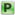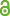Hauptmenü
• Autor
• Roeschel, Otto
• TitelPolygons and iteratively regularizing affine transformations
• Datei
• DOI10.1007/s13366-016-0313-7
• Persistent Identifier
• Erschienen inBeiträge zur Algebra und Geometrie / Contributions to Algebra and Geometry
• Band58
• Erscheinungsjahr2017
• Heft1
• Seiten69-79
• ISSN2191-0383
• Zugriffsrechte• AbstractWe start with a generic planar n-gon $$Q_0$$ with veritices $$q_{j,0}(j=0, \dots, n-1)$$ and fixed reals $$u, v, w \in \mathbb{R}$$ with $$u + v + w = 1$$. We iteratively define n-gons $$Q_k$$ of generation $$k \in \mathbb{N}$$ with vertices $$q_{j,k} (j = 0, \dots, n-1)$$ via $$q_{j,k} := u\ q_{j,k-1} + v\ q_{j+1,k-1} + w\ q_{j+2,k-1}$$. We are able to show that this affine iteration process for general input data generally regularizes the polygons in the following sense: There is a series of affine mappings $$\beta_k$$ such that the sums $$\Delta_k$$ of the squared distances between the vertices of $$\beta_k(Q_k)$$ and the respective vertices of a given regular prototype polygon P form a null series for $$k \longrightarrow \infty$$.